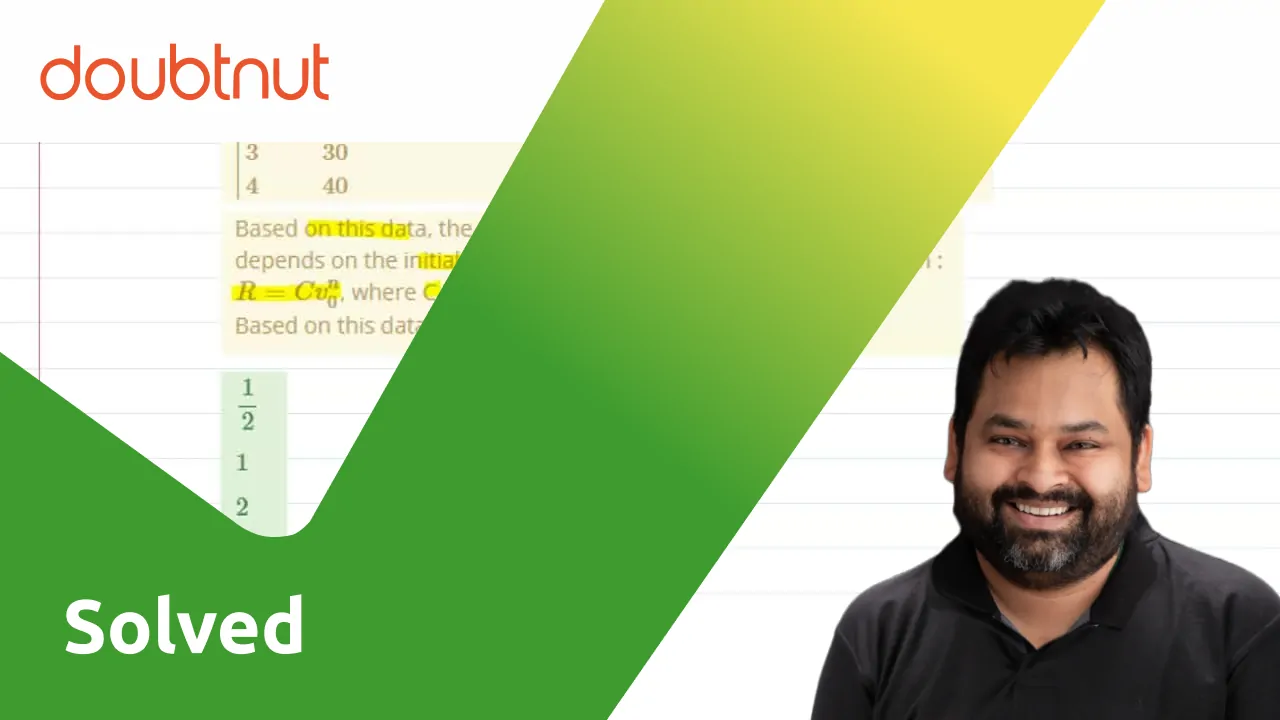Doubtnut App - Get 50 Lakh+ video solutions better than GoogleInstall Now

# A student performs an experiment to determine how the range of a ball depends on the velocity with which it is projected. The "range" is the distance between the points where the ball lends and from where it was projected, assuming it lands at the same height from which it was projected. It each trial, the student uses the same baseball, and launches it at the same angle. Table shows the experimental results. ∣∣ ∣ ∣ ∣ ∣ ∣∣TrailLaunch speed(m/s)Range(m)110822031.833070.7440122.5∣∣ ∣ ∣ ∣ ∣ ∣∣ Based on this data, the student then hypothesizes that the range, R, depends on the initial speed v0 according to the following equation : R=Cvn0, where C is a constant and n is another constant. Based on this data, the best guess for the value of n is :-Solution in Gujrati

A

12

B

1

C

2

D

3

Text Solution
Verified by Experts

## R=Cvn0 Putting data from table: 8=C×10n ⇒31.8=C×20n⇒31.88=3.9≅4=2n⇒n=2